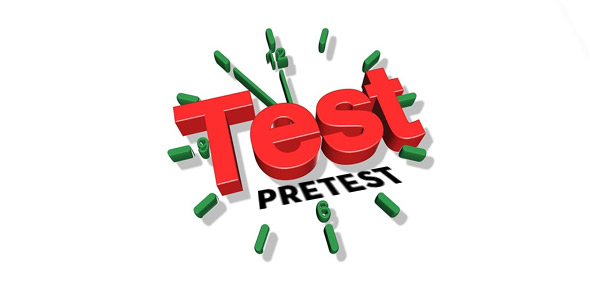# Rates And Ratios Pretest

10 Questions | Total Attempts: 85SettingsAnswer these questions as well as you can.

• 1.
Convert 3.6km to m.
• 2.
2 1/7 as an improper fraction is 23/7
• A.

True

• B.

False

• 3.
0.52517 rounded to 2 decimal places is:
• A.

0.53

• B.

0.52

• 4.
Simplify the following: 36/48
• A.

18/24

• B.

9/12

• C.

3/4

• 5.
Convert 2000mm to cm
• A.

20cm

• B.

200cm

• C.

2cm

• D.

2m

• 6.
3.899 rounded to 2 decimal places is _____
• 7.
If 2/3 = 8/x, what is x?
• 8.
If x/2 = 21/6, what is x?
• A.

3

• B.

1

• C.

7

• 9.
30% of 150 is ______
Related TopicsBack to top